# How to multiply cells in Excel with formula examples

The tutorial explains how to multiply in Excel by using the multiplication symbol and functions, how to create a formula for multiplying cells, ranges or entire columns, how to multiply and sum, and more.

While there is no universal multiplication formula in Excel, there exist a few different ways to multiply numbers and cells. The below examples will teach you how to write a formula best suited for your specific task.

## Multiply in Excel by using multiplication operator

The easiest way to do multiplication in Excel is by using the multiply symbol (*). With this approach, you can quickly multiply numbers, cells, entire columns and rows.

### How to multiply numbers in Excel

To make the simplest multiplication formula in Excel, type the equals sign (=) in a cell, then type the first number you want to multiply, followed by an asterisk, followed by the second number, and hit the Enter key to calculate the formula.

For example, to multiply 2 by 5, you type this expression in a cell (with no spaces): `=2*5`

As shown in the screenshot below, Excel allow performing different arithmetic operations within one formula. Just remember about the order of calculations (PEMDAS): parentheses, exponentiation, multiplication or division whichever comes first, addition or subtraction whichever comes first.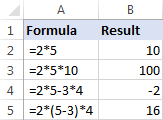### How to multiply cells in Excel

To multiply two cells in Excel, use a multiplication formula like in the above example, but supply cell references instead of numbers. For example, to multiply the value in cell A2 by the value in B2, type this expression:

`=A2*B2`

To multiply multiple cells, include more cell references in the formula, separated by the multiplication sign. For example:

`=A2*B2*C2`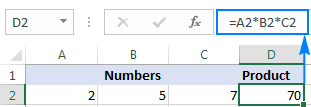### How to multiply columns in Excel

To multiply two columns in Excel, write the multiplication formula for the topmost cell, for example:

`=A2*B2`

After you've put the formula in the first cell (C2 in this example), double-click the small green square in the lower-right corner of the cell to copy the formula down the column, up to the last cell with data: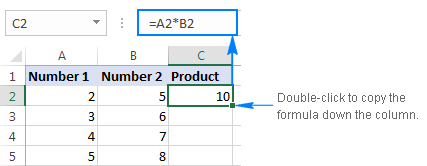Due to the use of relative cell references (without the \$ sign), our Excel multiply formula will adjust properly for each row: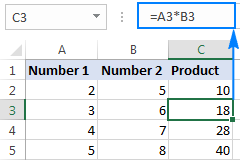In my opinion, this is the best but not the only way to multiply one column by another. You can learn other approaches in this tutorial: How to multiply columns in Excel.

### How to multiply rows in Excel

Multiplying rows in Excel is a less common task, but there is a simple solution for it too. To multiply two rows in Excel, just do the following:

1. Insert a multiplication formula in the first (leftmost) cell.

In this example, we multiply values in row 1 by the values in row 2, beginning with column B, so our formula goes as follows: `=B1*B2`

2. Select the formula cell, and hover the mouse cursor over a small square at the lower right-hand corner until it changes to a thick black cross.
3. Drag that black cross rightward over the cells where you want to copy the formula.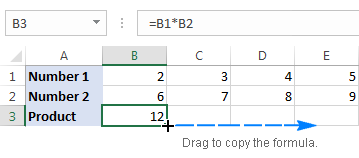As with multiplying columns, the relative cell references in the formula change based on a relative position of rows and columns, multiplying a value in row 1 by a value in row 2 in each column: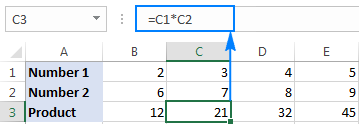## Multiply function in Excel (PRODUCT)

If you need to multiply multiple cells or ranges, the fastest method would be using the PRODUCT function:

PRODUCT(number1, [number2], …)

Where number1, number2, etc. are numbers, cells or ranges that you want to multiply.

For example, to multiply values in cells A2, B2 and C2, use this formula:

`=PRODUCT(A2:C2)`

To multiply the numbers in cells A2 through C2, and then multiply the result by 3, use this one:

`=PRODUCT(A2:C2,3)`

The screenshot below shows these multiplication formulas in Excel: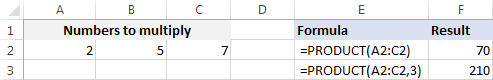## How to multiply by percentage in Excel

To multiply percentages in Excel, do a multiplication formula in this way: type the equals sign, followed by the number or cell, followed by the multiply sign (*), followed by percentage.

In other words, make a formula similar to these:

• To multiply a number by percentage: `=50*10%`
• To multiply a cell by percentage: `=A1*10%`

Instead of percentages, you can multiply by a corresponding decimal number. For example, knowing that 10 percent is 10 parts of a hundred (0.1), use the following expression to multiply 50 by 10%: `=50*0.1`

As shown in the screenshot below, all three expressions yield the same result: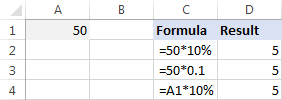## How to multiply a column by a number in Excel

To multiply a column of numbers by the same number, proceed with these steps:

1. Enter the number to multiply by in some cell, say in A2.
2. Write a multiplication formula for the topmost cell in the column.

Assuming the numbers to be multiplied are in column C, beginning in row 2, you put the following formula in D2:

`=C2*\$A\$2`

It is important that you lock the column and row coordinates of the cell with the number to multiply by to prevent the reference from changing when you copy the formula to other cells. For this, type the \$ symbol before the column letter and row number to make an absolute reference (\$A\$2). Or, click on the reference and press the F4 key to change it to absolute.

3. Double-click the fill handle in the formula cell (D2) to copy the formula down the column. Done!

As you can see in the screenshot below, C2 (relative reference) changes to C3 when the formula is copied to row 3, while \$A\$2 (absolute reference) remains unchanged: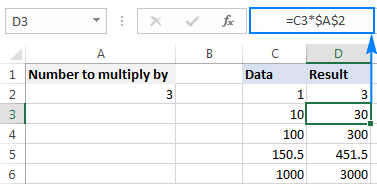If the design of your worksheet does not allow an additional cell to accommodate the number, you can supply it directly in the formula, e.g.: `=C2*3`

You can also use the Paste Special > Multiply feature to multiply a column by a constant number and get the results as values rather than formulas. Please check out this example for the detailed instructions.

## How to multiply and sum in Excel

In situations when you need to multiply two columns or rows of numbers, and then add up the results of individual calculations, use the SUMPRODUCT function to multiply cells and sum products.

Supposing you have prices in column B, quantity in column C, and you want to calculate the total value of sales. In your math class, you'd multiply each Price/Qty. pair individually and add up the sub-totals.

In Microsoft Excel, all these calculations can be done with a single formula:

`=SUMPRODUCT(B2:B5,C2:C5)`

If you wish, you can check the result with this calculation:

`=(B2*C2)+(B3*C3)+(B4*C4)+(B5*C5)`

And make sure the SUMPRODUCT formula multiplies and sums perfectly: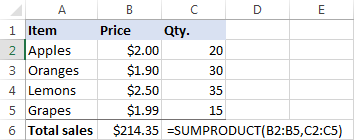## Multiplication in array formulas

In case you want to multiply two columns of numbers, and then perform further calculations with the results, do multiplication within an array formula.

In the above data set, another way to calculate the total value of sales is this:

`=SUM(B2:B5*C2:C5)`

This Excel Sum Multiply formula is equivalent to SUMPRODUCT and returns exactly the same result (please see the screenshot below).

Taking the example further, let's find an average of sales. For this, just use the AVERAGE function instead of SUM:

`=AVERAGE(B2:B5*C2:C5)`

To find the largest and smallest sale, use the MAX and MIN functions, respectively:

`=MAX(B2:B5*C2:C5)`

`=MIN(B2:B5*C2:C5)`

To complete an array formula properly, be sure to press the Ctrl + Shift + Enter combination instead of Enter stroke. As soon as you do this, Excel will enclose the formula in {curly braces}, indicating it's an array formula.

The results might look something similar to this: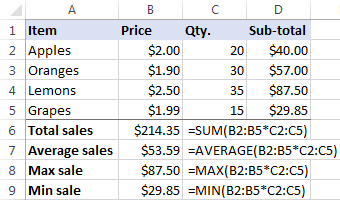That's how you multiply in Excel, it doesn't take a rocket scientist to figure it out :) To have a closer look at the formulas discussed in this tutorial, feel free to download our sample Excel Multiplication workbook.

## How to quickly do any calculations in Excel

If you are a novice to Excel and are not comfortable with multiplication formulas yet, our Ultimate Suite will make things a lot easier for you. Among 70+ cute features, it provides the Calculation tool that can perform all basic mathematic operations, including multiplication, in a mouse click. Let me show you how.

Supposing you have a list of net prices and you want to know the corresponding VAT amount. No big deal if you know how to calculate percentages in Excel. If you don't, have the Ultimate Suite do the job for you:

1. Copy the prices to the VAT column. You need to do this because you don't want to override the original values in the Price column.
2. Select the copied prices (C2:C5 in the screenshot below).
3. Go to the Ablebits tools tab > Calculate group, and do the following:
• Select the percentage symbol (%) in the Operation box.
• Type the desired number in the Value box.
• Click the Calculate button.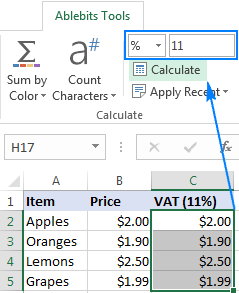That's all there is to it! You will have the percentages calculated in a heartbeat: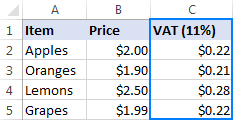In a similar manner, you can multiply and divide, add and subtract, calculate percentages, and more. All you have to do is select an appropriate operator, for example the multiply symbol (*):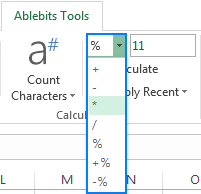To perform one of the recent calculations to another range or column, just click the Apply Recent button, and pick the operation: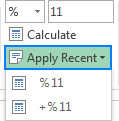The results of all calculations done with the Ultimate Suite are values, not formulas. So, you are free to move or copy them to another sheet or workbook without worrying about updating formula references. The calculated values will remain intact even if move or delete the original numbers.

I thank you for reading and hope to see you on our blog next week!

## You may also be interested in

1. I am trying to multipy a range of cells by .127, when a cell contains a certain value, and total all the products. I have a very long and cumbersome series of If statements, but I know there has to be a way to write this more simply. Here is my current formula:

=(IF(I16="GXP",K16*\$A\$3*\$B\$1,0)+IF(I17="GXP",K17*\$A\$3*\$B\$1,0)+IF(I18="GXP",K18*\$A\$3*\$B\$1,0)+IF(I19="GXP",K19*\$A\$3*\$B\$1,0)+IF(I20="GXP",K20*\$A\$3*\$B\$1,0)+IF(I21="GXP",K21*\$A\$3*\$B\$1,0)+IF(I22="GXP",K22*\$A\$3*\$B\$1,0)+IF(I37="GXP",K37*\$A\$3*\$B\$1,0)+IF(I38="GXP",K38*\$A\$3*\$B\$1,0)+IF(I39="GXP",K39*\$A\$3*\$B\$1,0)+IF(I40="GXP",K40*\$A\$3*\$B\$1,0)+IF(I41="GXP",K41*\$A\$3*\$B\$1,0)+IF(I42="GXP",K42*\$A\$3*\$B\$1,0)+IF(I43="GXP",K43*\$A\$3*\$B\$1,0)+IF(I44="GXP",K44*\$A\$3*\$B\$1,0)+IF(I45="GXP",K45*\$A\$3*\$B\$1,0)+IF(I46="GXP",K46*\$A\$3*\$B\$1,0)+IF(I47="GXP",K47*\$A\$3*\$B\$1,0)+IF(I48="GXP",K48*\$A\$3*\$B\$1,0)+IF(I51="GXP",K51*\$A\$3*\$B\$1,0)+IF(I53="GXP",K53*\$A\$3*\$B\$1,0))+B45

2. Please How could I work this out
E.g
259+576

3. Hello-

I'm having trouble with a formula. I need to divide a number by an addition problem. I am trying to eliminate the DIV error, when the cells are 0. How can I write the formula to avoid the error? I have tried =IFERROR((C26/(C18+C20) but it does not work. The formula works when I write the equation backwards as =IFERROR((C18+C20)/C26)), but I get an incorrect answer. I really need your help.

Thanks

4. Im trying to figure a way to calculate mileage distrubted. for Ex I have column A and it has 30 different millage ranges which all some up to Row (A31) 170,000. and the total budget is \$20,000. I know how to get the average per mile which would be in row (A34) I put SUM(20,000/A31) and it gives me X
Now in column B I want to multiple each row by (A34) which looks like this =SUM (A1*A34) next is =SUM(A2*A34) but then I try to drag it down it keeps changing it to a different Cell From (A34). Can you help

5. Hi, can anyone help me with this formula I need to do a lot of them and it takes ages to do it manually but I don't know the quick way.
=SUMPRODUCT(J32*D32)+(J33*D33)+(J37*D37)+(J34*D34)+(J35*D35)+(J36*D36)+(J38*D38)+(J39*D39)+(J340*D40)+(J41*D41)+(J42*D42)+(J43*D43)+(J44*D44)+(J45*D45)+(J46*D46)+(J47*D47)+(J48*D48)+(J49*D49)+(J50*D50)+(J51*D51)+(J52*D52)+(J53*D53)+(J54*D54)+(J55*D55)+(J56*D56)+(J57*D57)+(J58*D58)+(J59*D59)

Thanks Becky

=SUMPRODUCT(D32:D59,J32:J59)

6. how to calculate this? ( 0.89+0.88+0.87+0.87+0.86+0.86+0.85+0.85+0.84)*7.06 +(0.83+0.83+0.82+0.82+0.81+0.81+0.80+0.80+0.79+0.78+0.78+0.77+0.77+0.76+0.76+0.75)7.57 /(0.89+0.88+0.87+0.87+0.86+0.86+0.85+0.85+0.84+0.83+0.83+0.82+0.82+0.81+0.81+0.80+0.80+0.79+0.78+0.78+0.77+0.77+0.76+0.76+0.75) My ans. 59.53 but actual ans. 7.3765

• Hi! Perhaps you just need to remember the order of mathematical operations and add parentheses.

7. Hello! I would like to ask for some help with multiplying by a range of numbers. I should mention I'm not very good with math so I don't know all the terms, but what I'm trying to do is have a single column with a range of numbers (like 1 through 8) being multiplied. An example of a problem i'm trying to translate into excel would be:
4*(1-65%)*125

• Hi!
In your formula, replace the number 1 with a reference to first cell of range. For example, 4*(A1-65%)*125.
Then copy that formula down the column.

8. I need to multiply three cells. first two cell has simple numbers but third cell has condition that
if number is <=99.99 then multiply by 1 and number above 99.99 multiply by 1.5 and add them both.
for example, if number is 112 then [(99.99*1)+(12.01*1.5)]*cell 1*cell 2
how do I write formula for this? TYA.

• Hi!
If you have conditions then use the IF function
Try this formula:

=IF(A1 > 99.99,(99.99+(A1-99.99)*1.5)*B1*C1),A1)

9. I have a problem calculating exact values. When I am calculating results are rounding off

Exact value required for B7*B4*C6 where
B4=25
B5=B1(input value)
B7= B5*10%
C5=B5*B4
C6= B3/C5

But the results are rounding to the nearest 500

Example:
B5= 890
B7= 89
C5= 22250
C6= 2
So, the results should be 89*2*25 = 4450 but it's coming as 5000.

• Hi!
I was unable to reproduce your issue. Check which number format is set in the cell. Set the cell format to General.

10. Dollar Rate 205

Rs2,200,000.00 Rs4,500,000.00 Rs9,800,000.00

\$10,731.71 \$21,951.22 \$47,804.88 0.20% 95.6097561 19600

If I changes the value/Dollar Rate the rest of value changed , but the after % Multiplication the final result/19600 could not changes
anyone can Help to Solve ?

11. I want to calculate the percentages of various amount like if i have 230,000 and 200,000 will get 0.5% and 30,000 will get 0.25%?

12. If Multiply using " =leftright "formula
150*150 in A1 Cell which is single cell
Let's have a look after applying formula in B1(reference cell is B1 where I want results
=LEFT(A1,3)*RIGHT(A1,3)
yes result is 22500 in b1,but what about I drag formula on down cell,if in next row A2 12*12 and formula automatically putted by draging but the result is" 0" it doesn't work cause chars or digit changed in A2 cell,if I change the formula manually in function bar =left(A2,2) replace 3 with 2, same way replace in=right( ,2) the result is 144
Exactly I need a single a formula which perform in any digits or chars,

• Hi!
Determine the position of the "*" character using the SEARCH function. Extract the text to the right and left of this position using the substring functions.

=LEFT(A1,SEARCH("~*",A1)-1)*MID(A1,SEARCH("~*",A1)+1,20)

13. Thank you so much!!

14. I have to calculate total number of days.
A1 cell has data 2.2.15 (ie.. 2 years.2 months,15 days)
Now, i want to convert this to total days with formula to calculate. And this formula to be applied for many cells

calculation = > 2*370+2*30+15=765 days.
Pl. share the formula to calcuate the same.

• Hello!
To extract three values from text, you can use these three formulas:

=LEFT(A2, SEARCH(".",A2)-1)
=MID(A2, SEARCH(".", A2) + 1, SEARCH(".", A2, SEARCH(".", A2)+1) - SEARCH(".", A2)-1)
=RIGHT(A2,LEN(A2) - SEARCH(".", A2, SEARCH(".", A2,1)+1))

You can find the examples and detailed instructions here: Excel substring: how to extract text from cell.
To split text into cells, you can also use the Text To Columns feature.

15. Hi,

I'm struggling to find the right formula to multiply units by rates.

I have different materials with different units and rates. Rates are depend on quantities.

Description Unit Quantity Rate 1 Rate 2 Rate 4 Rate 5 Price
path m (QB) 50 11 13 13.5 14
wall m2 (QB) 35
wood m 10
paint m2 12

Unit "Rate 1
(not exceeding)" "Rate 2
(not exceeding)" "Rate 3
(not exceeding)" "Rate4
(exceeding)"
day
h
m (QB) 10 50 200 200
m
m2 (QB) 10 50 150 15
m2

• Hi!
This is a complex solution that cannot be found with a single formula. If you have a specific question about the operation of a function or formula, I will try to answer it.

• Hello how can I calculate cell 1*cell2 +cell3*cell4...../ 11?

16. I have to know the excel formula for multiply more the three currency with one column

for e.g.

A
USD 100
B
AED 100
C
Eur 100

D
Exchange Rate
E
If the rate of USD is there I have to calculate with D exchange rate
If the value of C is AED is there then I have to Calculate with D exchange rate

How should I multiply this with excel formula?
Pls help

• INVOICE CURRENCY VALUE IN USD
USD INR AED EX RATE
\$5,987.27 1.00 \$-

• Hi!
If I understand you correctly, then in one cell D1 you want to write 3 exchange rate values. This is not possible in Excel. You can use the VLOOKUP function to select the desired exchange rate based on the currency. If there are a total of 3 exchange rates, write them down in separate cells (for example, F1:F3), and use nested IF function:

=IF(A1 < > "",F1,F(B1 < > "",F2,F(C1 < > "",F3,"")))

17. Can excel complete a calculation for multiples of say 7. So you start obviously with 7, then have it auto generate out 14, 21, 28, 35, etc? And control its start and end number so if you wanted to start at say 336 and have it provide multiples of 7 generated out up to at least 1,000?

18. Hi,
How do I multiply a whole row by the same number?
Eg. All of row B needs to be multiplied by -1.
How would I do this
Thank you

19. If you have a columb of numbers, how can you set excel to multiply a specific value inside the column. Example every time a Columb has a consecutive 0 in it I would like to multiple by (\$100 or the growing balance by 2 until the next number isnt 0). Thanks for input.

• Hi!

20. Hi,

I am trying work out commissions and by typing 'Y' next to the commission type. Buy i would like to know how much commission is accumulated at the bottom. Is there a way to create a formula that times' the amount of 'Y' but an amount?

Thanks :)

• Hi!
The information you provided is not enough to understand your case and give you any advice, sorry. Please specify what you were trying to find, what formula you used and what problem or error occurred. Give an example of the source data and the expected result.

21. Hi,

How can I count a sum of multiple values where some have different percentage multipliers. E.g

A1:A1000 have different numeric values. The sum of these values is a percentage (eg. 60% x sum of values). BUT some specific values e.g. 89\$ should be calculated with another percentage (70%.) How do I create a formula that calculates all values with 60% except 89 dollar values (with 70%) and adds them all up?

22. How do make the column multiply by itself without having to input the formula?

• Hi!
This can be done with a VBA macro.

23. I'm looking for a formula that will multiply a cell by .25 but only the first 50 counted in that cell. So if the cell is say 65 I want it to only multiply 50 by .25.

• Hi!
Use condition with IF function:

=IF(A1<=50,A1*0.25,50*0.25)

24. Trying to multiply the following cells with the following numbers in the cell. Example as follows...

Cell.. AB1 (has the number 231 in it)
Cell.. AC1 (has the number 2.529 in it)

The 231 is fuel and \$2.529 is the cost per gallon and I need the total costs of the fuel

25. How to multiply rows with different multiplier

eg:Value X
Multipliers - 1,2,3,4,5
looking formula for x*1,x*2,x*3.....

• Hi!
What do you want to calculate exactly? Your question is not entirely clear, please specify.

26. I want to one formula people age.
If current time and date in one box fix.
One column is date of birth. One column vale is either death or alive. If people die results show zero.

27. A B C D E
1 SAKO Portier Cleaning %
3 Thomas 15.2
4 Jack 11.0
5 John 11.0
6 Smith 12.2
7 Jef 8.4
8 David 20.6
9 Total 3400 5500 7300 100

Hi
Could you please provide me with an excel formula to fill out the above Excel matrix by distributing the items in total row among the persons by using the percentage stated in the column. Many thanks for your kind cooperation

• Hello!
The formula in C1 might be something like this.

=B1*\$C\$9

Copy it down the column.

28. If there is only one value at the time of multiplication, then there will be a specific mark in the cell, How to do it in Excel?

29. =IF(L20=9,(M23*0.9+K14*0.15))

Didn't seem to post the formula correctly

30. I have 2 formulas, they work by them selves but when I try and add them together it will not work, What am I doing wrong.

=IF(L20=9,M23*0.9+K14*0.15)

Thanks

31. how to multiply B3?

32. 19.46 * 8000 is giving me 155,652.91 but the real answer is 155,680. Why is excel giving me this number?

• Hello!
I assume that 19.46 is the result of calculating the formula, and not entering this value manually. If you increase the number of decimal places in the number format, you will see that this is not 19.46 but 19.45661375
You can use the ROUND function in the formula so that the number on the screen does not differ from the real number.

33. Here is my example: My balance is \$1045.00. My rate is 4.5%. My minimum payment is \$45.00. I plan to add an additional monthly extra payment of \$400.00. I can figure this out on Excel spreadsheet by using a running balance, monthly interest, and the monthly payment. I want to be able to calculate this on paper to get the future amount owed or total interest paid without calculator. Thank you in advance.

34. I need to multiply one cell by another, repeatedly where only one changes. So, I want C3 * D2, C4*D2, C5*D2, but when I put in the first formula and drag down the column, it gives me C3*D2, C4*D3, C5*D4 -- it changes the second number. I have tried putting a \$ before the D value =PRODUCT(C3*\$D2) but it does not help. I want the D2 to remain constant and each C to multiply by the D2 number.

35. Total \$784,802.74

Contracting fee 15% + gst \$117,720.41 -need this formula to also include the GST IE: =+B55*15% +(B57*5%) - wont let me do this in current column. Circular reference error if i do this? The amount should be \$123,606.43

Estimated - New Budget Total \$902,523.15 This total would then be: \$908409.17

36. Hello,

I want to calculate:

(1+Q13*\$N\$2)+PRODUCT(1+Q13:Q14*\$N\$2)+PRODUCT(1+Q13:Q15*\$N\$2)+... +PRODUCT(1+Q13:Q33*\$N\$2)
is there any shorter way I dont need to write them all?

• Hi,
Unfortunately, without seeing your data it is difficult to give you any advice. Could you please describe it in more detail? What result do you want to get? Please provide me with an example of the source data and the expected result.